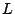# Function field zeta-functions

Much of our understanding about the connection between-functions and random matrix theory comes from analogies with function fields, for in the function fields case it is possible to prove many of the results which are only conjectured for number fields. This is described in the book of Katz and Sarnak [ MR 2000b:11070].

The analogy between the two cases is not perfect (or at least, not perfectly understood). For example, it is not known what should play the role of monodromy in the number field case. Another example is the proof of the Riemann hypothesis. In the function field case the proof makes use of high tensor powers, so it would be desirable to understand the analogue of this in the number field case.

In this section we address questions relating to function fields, as well as the analogy between function fields and number fields.

Back to the main index for L-functions and Random Matrix Theory.